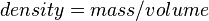Density facts for kids

Kids Encyclopedia Facts
(Redirected from Dense)

Density is a measurement that compares the amount of matter an object has to its volume. An object with a lot of matter in a certain amount of volume has high density. An object with a little matter in the same amount of volume has a low density.

Temperature has an effect on density. When the temperature of a substance increases, density decreases because the atoms are moving all around the place having less matter in one space. When the temperature of a substance decreases, the density increases because the atoms move slower making the atoms stay in one place. Many substances follow this rule, but there are exceptions. Water is one such exception. When water freezes, it makes ice that is less dense than liquid water.

Fresh water is often used as a standard of relative density. This is called specific gravity.

The most common SI units for density are g/cm3 and kg/m3. When the numerator is much larger than the denominator, that means the substance has a higher density. When the denominator is much larger than the numerator, the substance has a lower density.

A formula to find out density is:$density = mass / volume$

Sometimes scientists use the idea of density to talk about other properties instead of mass. They're trying to describe how much of a property can be found in a specific piece of what they are looking at. For example, population density is how many people live within the same amount of space. The population density in the city is higher than the country side because there are more people living closer to each other in the city. In computers, storage density is how much data can fit on a data storage device in relation to its physical size. A Blu-ray disc has a higher storage density than a DVD which has a higher storage density than a CD, even though they all have almost exactly the same volume.

Images for kidsDensity Facts for Kids. Kiddle Encyclopedia.